Integration by specific formulaes - Method 10

Chapter 7 Class 12 Integrals
Concept wise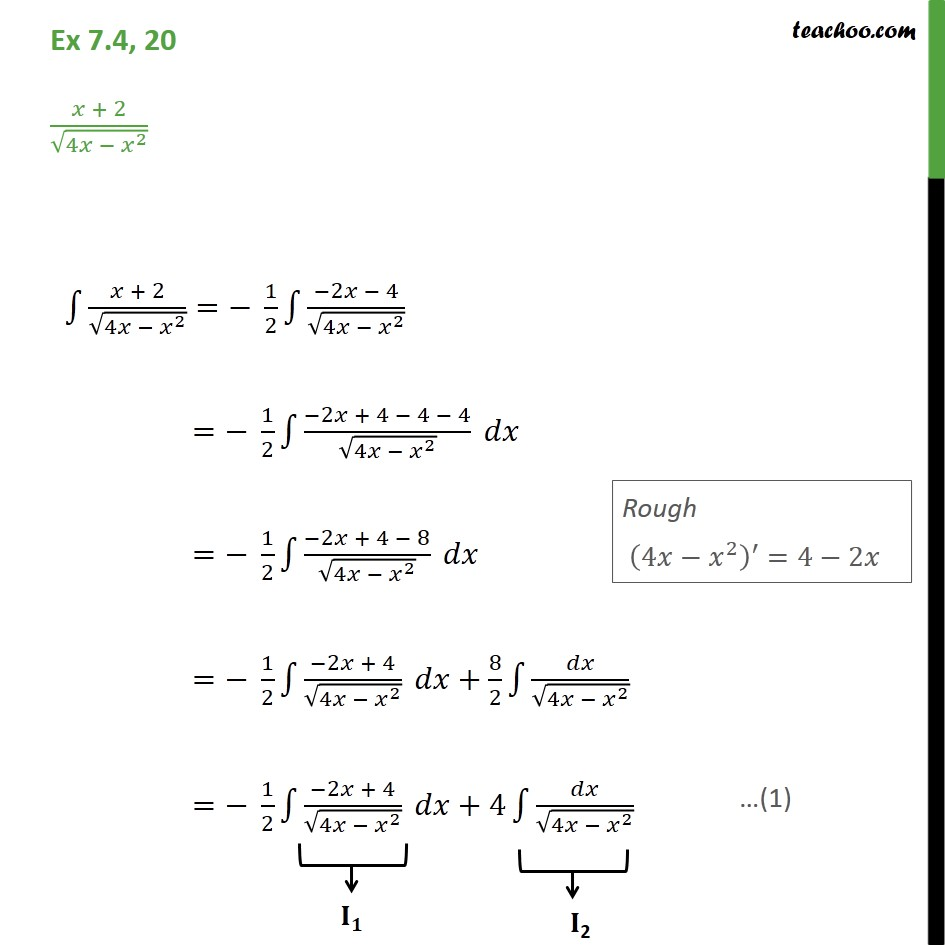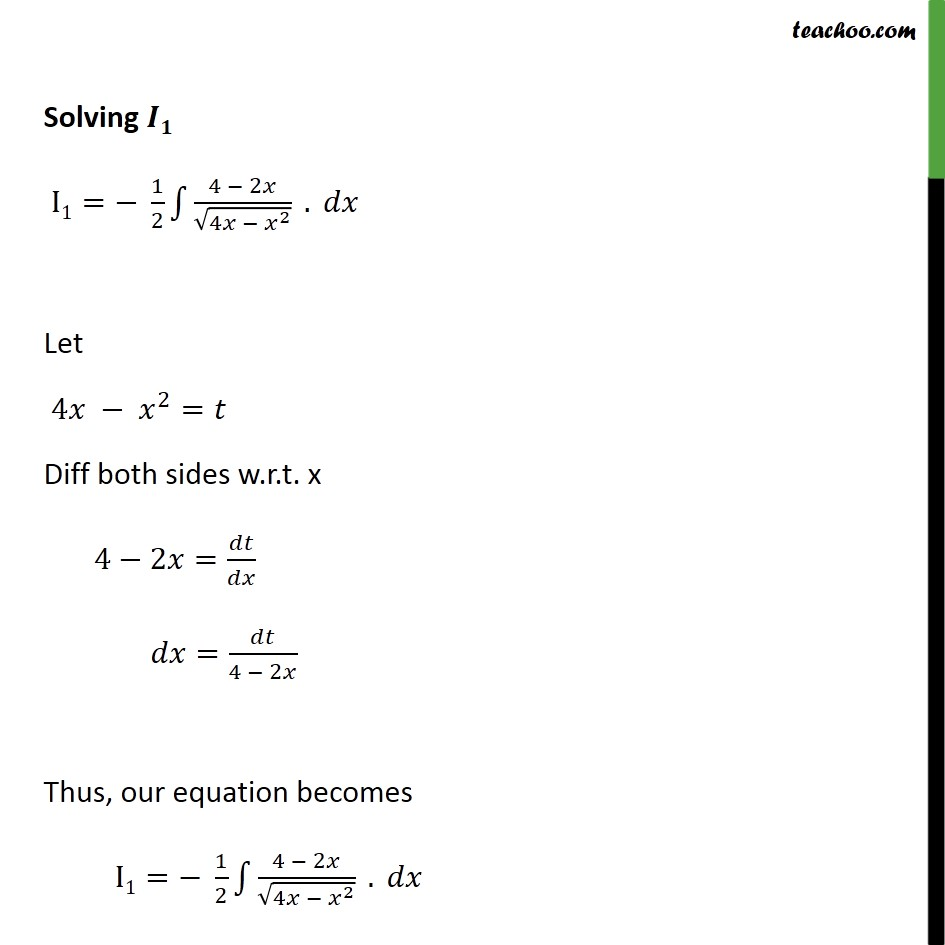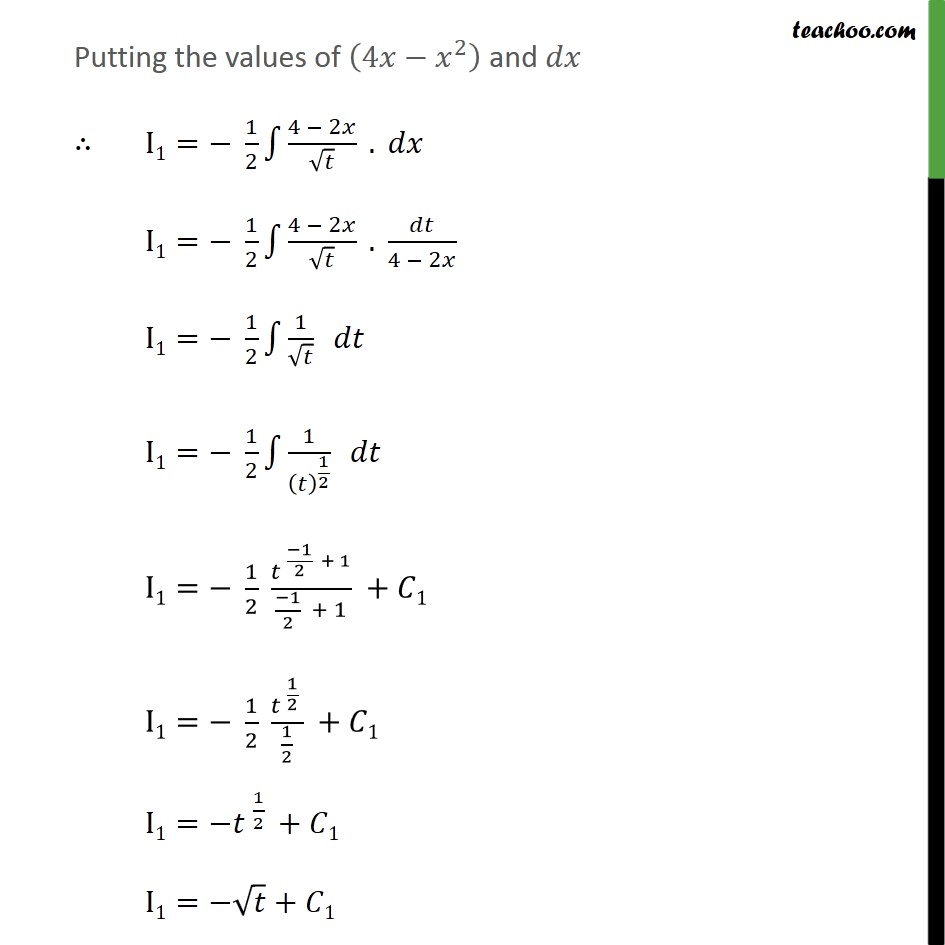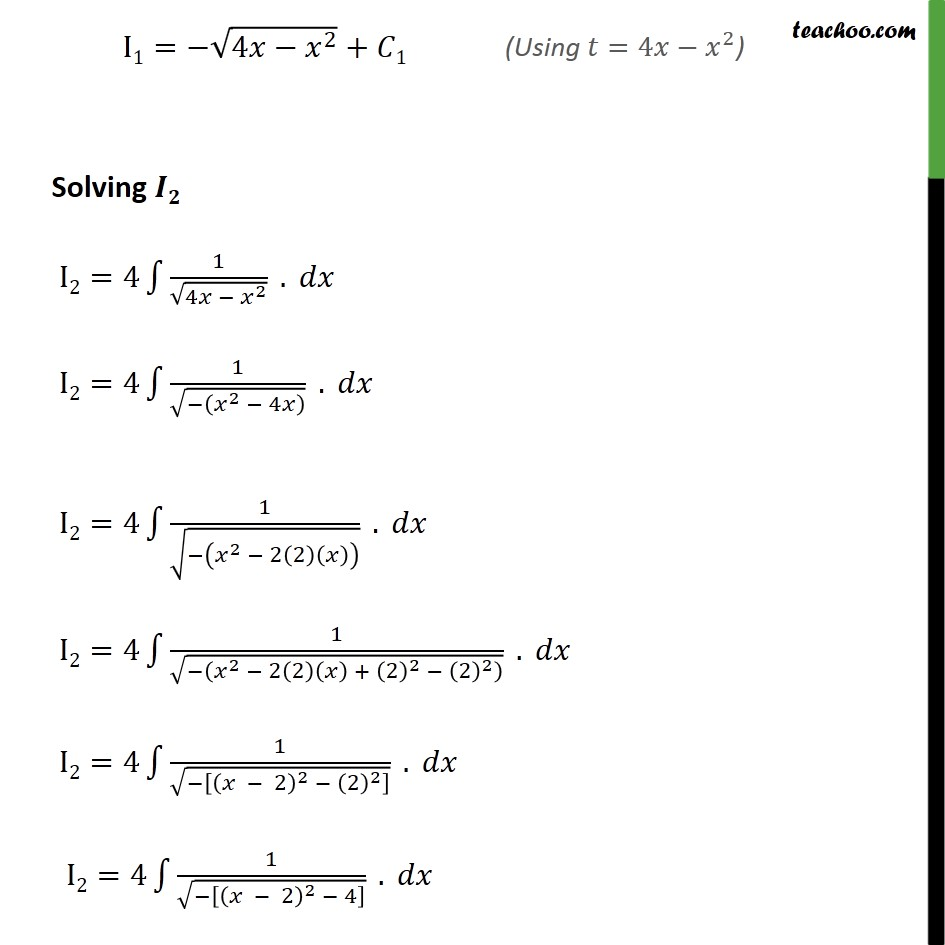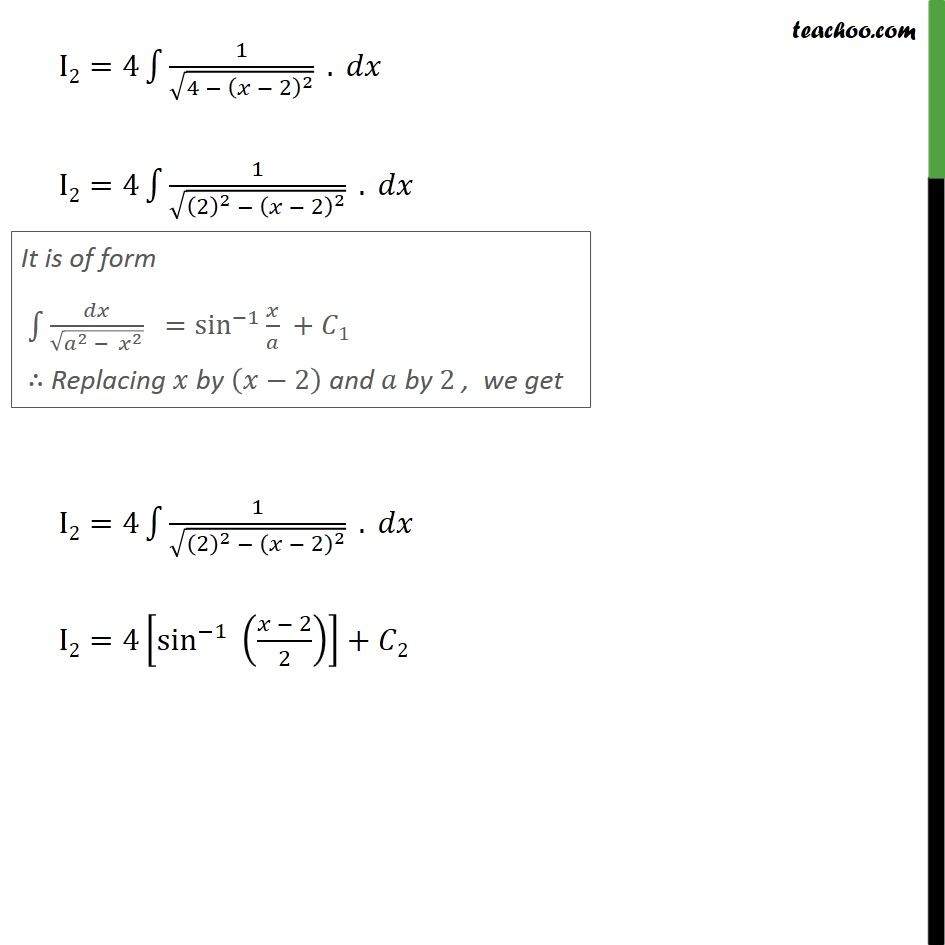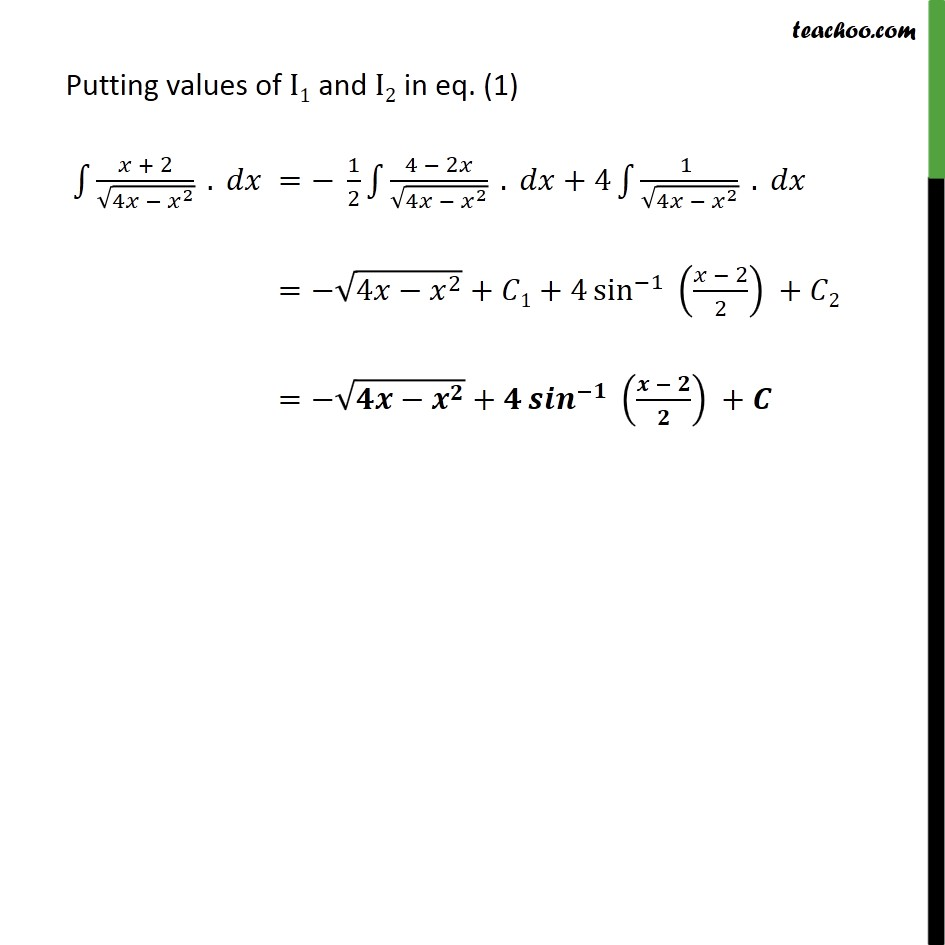Introducing your new favourite teacher - Teachoo Black, at only ₹83 per month

### Transcript

Ex 7.4, 20 ( + 2)/ (4 ^2 ) 1 ( + 2)/ (4 ^2 )= 1/2 1 ( 2 4)/ (4 ^2 ) = 1/2 1 ( 2 + 4 4 4)/ (4 ^2 ) = 1/2 1 ( 2 + 4 8)/ (4 ^2 ) = 1/2 1 ( 2 + 4)/ (4 ^2 ) +8/2 1 / (4 ^2 ) = 1/2 1 ( 2 + 4)/ (4 ^2 ) +4 1 / (4 ^2 ) Solving I1= 1/2 1 (4 2 )/ (4 ^2 ) . Let 4 ^2= Diff both sides w.r.t. x 4 2 = / = /(4 2 ) Thus, our equation becomes I1= 1/2 1 (4 2 )/ (4 ^2 ) . Putting the values of (4 ^2 ) and I1= 1/2 1 (4 2 )/ . I1= 1/2 1 (4 2 )/ . /(4 2 ) I1= 1/2 1 1/ I1= 1/2 1 1/( )^(1/2) I1= 1/2 ^(( 1)/2 + 1)/(( 1)/2 + 1) + 1 I1= 1/2 ( ^(1/2 ))/(1/2) + 1 I1= ^(1/2 )+ 1 I1= + 1 I1= (4 ^2 )+ 1 Solving I2=4 1 1/ (4 ^2 ) . I2=4 1 1/ ( ( ^2 4 ) ) . I2=4 1 1/ ( ( ^2 2(2)( )) ) . I2=4 1 1/ ( ( ^2 2(2)( ) + (2)^2 (2)^2 ) ) . I2=4 1 1/ ( [( 2)^2 (2)^2 ] ) . I2=4 1 1/ ( [( 2)^2 4] ) . I2=4 1 1/ (4 ( 2)^2 ) . I2=4 1 1/ ((2)^2 ( 2)^2 ) . I2=4 1 1/ ((2)^2 ( 2)^2 ) . I2=4[ sin^( 1) (( 2)/2) ]+ 2 Putting values of I1 and I2 in eq. (1) 1 ( + 2)/ (4 ^2 ) . = 1/2 1 (4 2 )/ (4 ^2 ) . +4 1 1" " / (4 ^2 ) . = (4 ^2 )+ 1+4 sin^( 1) (( 2)/2) + 2 = ( ^ )+ ^( ) (( )/ ) +